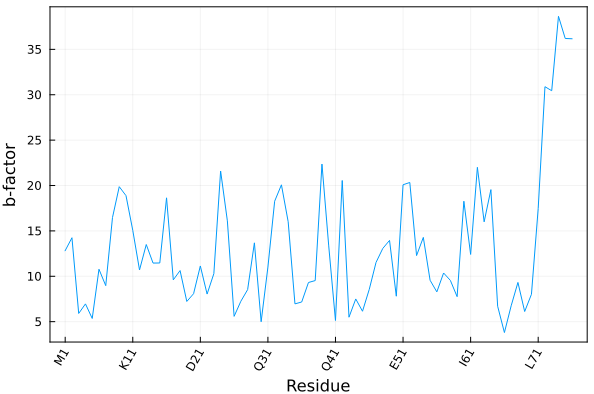# Some auxiliary functions to quickly retrieve some data

## Get the protein sequence

To obtain a list of the residue names of the protein with three- and one-letter codes, use

julia> seq = getseq("file.pdb")
76-element Vector{String}:
"V"
"K"
⋮
"R"
"G"


Use getseq(atoms,code=2) to get the sequence as three-letter residue codes, or code=3 to get full natural-aminoacid names, like "Alanine", "Proline", etc.

Note

If there is some non-standard protein residue in the sequence, inform the getseq function by adding a selection:

julia> getseq("file.pdb","protein or resname NEW")
77-element Vector{String}:
"V"
"N"
⋮
"R"
"G"

By default the selection will only return the sequence of natural amino acids.

The getseq function can of course be used on an Atom list, accepts selections as the last argument, as well as the reading and writing functions:

atoms = readPDB("file.pdb")
seq = getseq(atoms,"chain A")


## Distance between sets of atoms

The distance between atoms, or sets of atoms, can be computed with the distance function. This function returns the minimum distance between the atoms of the sets involved. For example:

julia> model = wget("1BSX");

julia> protein = select(model,"protein");

julia> ligand = select(model,"resname T3");

julia> distance(protein,ligand)
2.7775834820937417


## Closest atoms and their distance

A function similar to the one above is closest, which returns the shortest distance between atoms but also the identity of the atom or pair of atoms that satisfy that shortest distance:

julia> model = wget("1BSX");

julia> protein = select(model,"protein");

julia> ligand = select(model,"resname T3");

julia> closest(ligand,protein)
(43, 3684, 2.7775834820937417)

julia> ligand
4037   O1      T3     B        2      512  -22.568   81.625    3.159 36.59  1.00     1       -      4041

julia> protein
3684  NE2     HIS     B      435      472  -21.539   82.145    5.686 44.44  1.00     1       -      3686

julia> distance(ligand,protein)
2.7775834820937417


## Obtain arrays with coordinates

All atoms:

julia> x = coor(atoms)
1463-element Vector{SVector{3, Float64}}:
[-9.229, -14.861, -5.481]
[-10.048, -15.427, -5.569]
[-9.488, -13.913, -5.295]
⋮
[5.772, -10.399, -8.044]
[6.408, -12.034, -8.343]
[6.017, -10.967, -9.713]


Or use selections to retrieve the coordinates of subsets of atoms:

C$\alpha$ coordinates:

julia> xCA = coor(protein,"name CA")
104-element Vector{SVector{3, Float64}}:
[-8.483, -14.912, -6.726]
[-5.113, -13.737, -5.466]
[-3.903, -11.262, -8.062]
⋮
7.836, -2.933, -6.873]
[4.414, -4.302, -7.734]
[4.134, -7.811, -6.344]
[3.244, -10.715, -8.603]


The coordinates are output as arrays of static arrays (more specifically, as a Vector{SVector{3,Float64}}, from StaticArrays).

## Maximum and minimum coordinates of the atoms

Use maxmin(atoms), or maxmin(atoms,"resname CA"), for example:

julia> m = maxmin(atoms,"chain A")

Minimum atom coordinates: xmin = [-41.5, -41.526, -41.517]
Maximum atom coordinates: xmax = [41.583, 41.502, 41.183]
Length in each direction: xlength = [83.083, 83.028, 82.7]


m is a structure containing the three vectors with minimum and maximum coordinates, and lengths.

## Residue tick labels for plots

Compat

The residue_ticks function requires at least PDBTools version 0.14.1.

The residue_ticks function provides a practical way to define tick labels in plots associated to an amino-acid sequence:

residue_ticks(
atoms::AbstractVector{<:Atom};
first=nothing, last=nothing, stride=1, oneletter=true
)

The function returns a tuple with residue numbers and residue names for the given atoms, to be used as tick labels in plots.

first and last optional keyword parameters are integers that refer to the residue numbers to be included. The stride option can be used to skip residues and declutter the tick labels.

If oneletter is false, three-letter residue codes are returned. Residues with unknown names will be named X or XXX.

### Example

Here we illustrate how to plot the average temperature factor of each residue of a crystallographic model as function of the residues.

julia> using PDBTools, Plots

julia> atoms = wget("1UBQ", "protein");

julia> residue_ticks(atoms; stride=10) # example of output
([1, 11, 21, 31, 41, 51, 61, 71], ["M1", "K11", "D21", "Q31", "Q41", "E51", "I61", "L71"])

julia> plot(
resnum.(eachresidue(atoms)), # x-axis: residue numbers
[ mean(beta.(res)) for res in eachresidue(atoms) ], # y-axis: average b-factor per residue
xlabel="Residue",
xticks=residue_ticks(atoms; stride=10), # here we define the x-tick labels
ylabel="b-factor",
xrotation=60,
label=nothing, framestyle=:box,
)

Produces the following plot: